Ex 3.6

Chapter 3 Class 10 Pair of Linear Equations in Two Variables (Term 1)
Serial order wise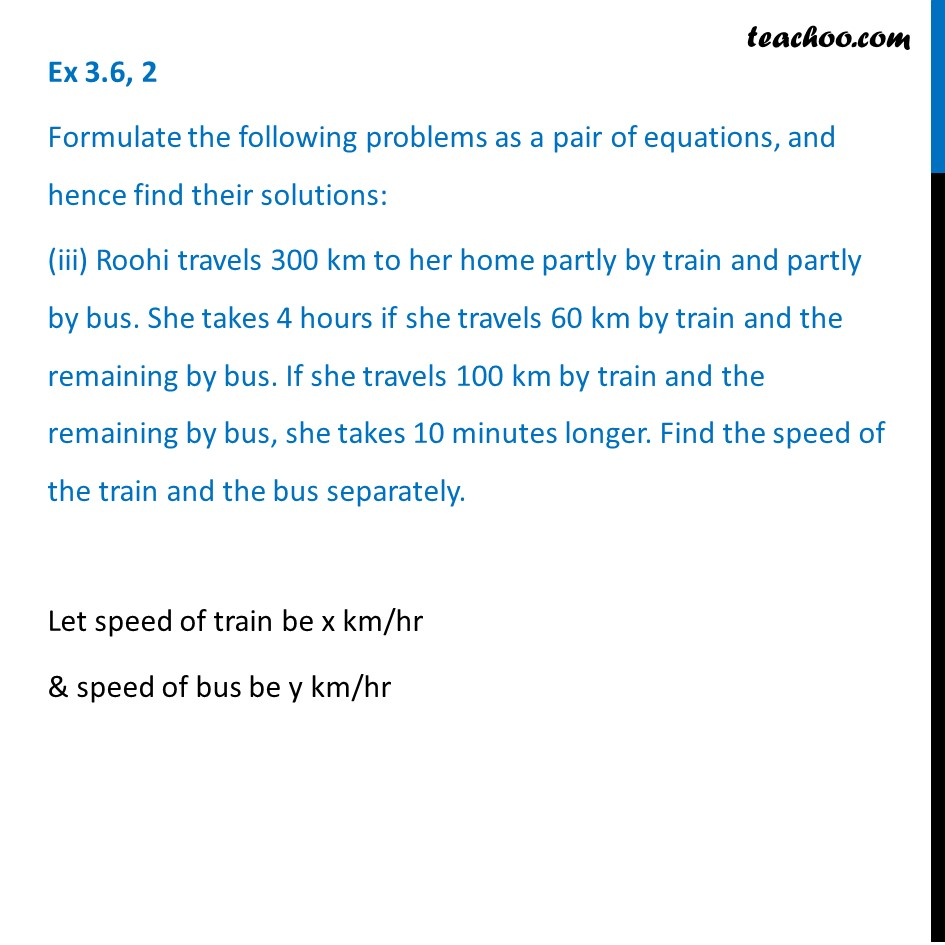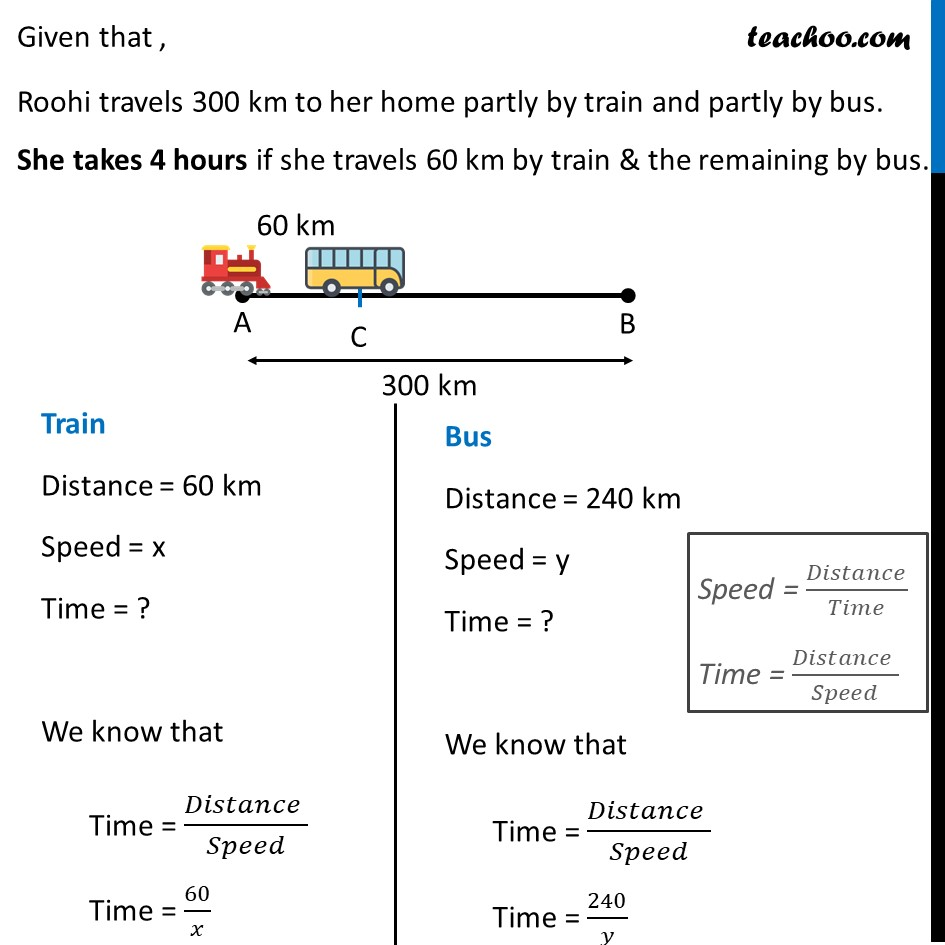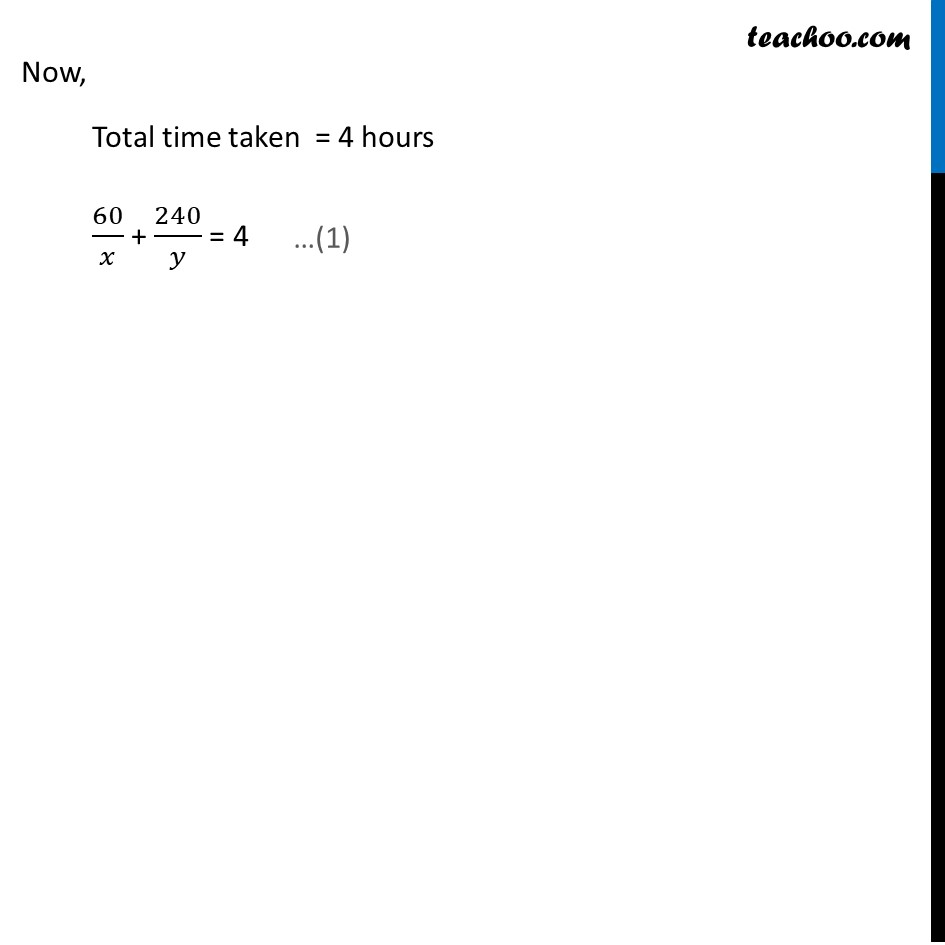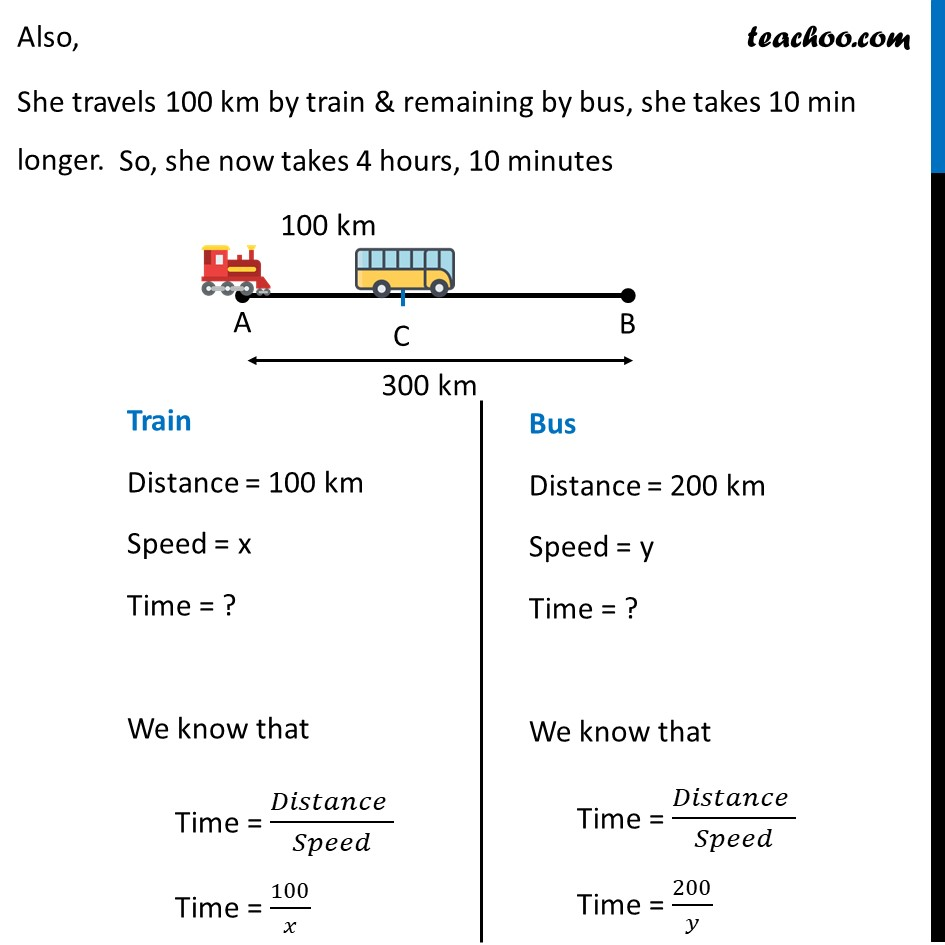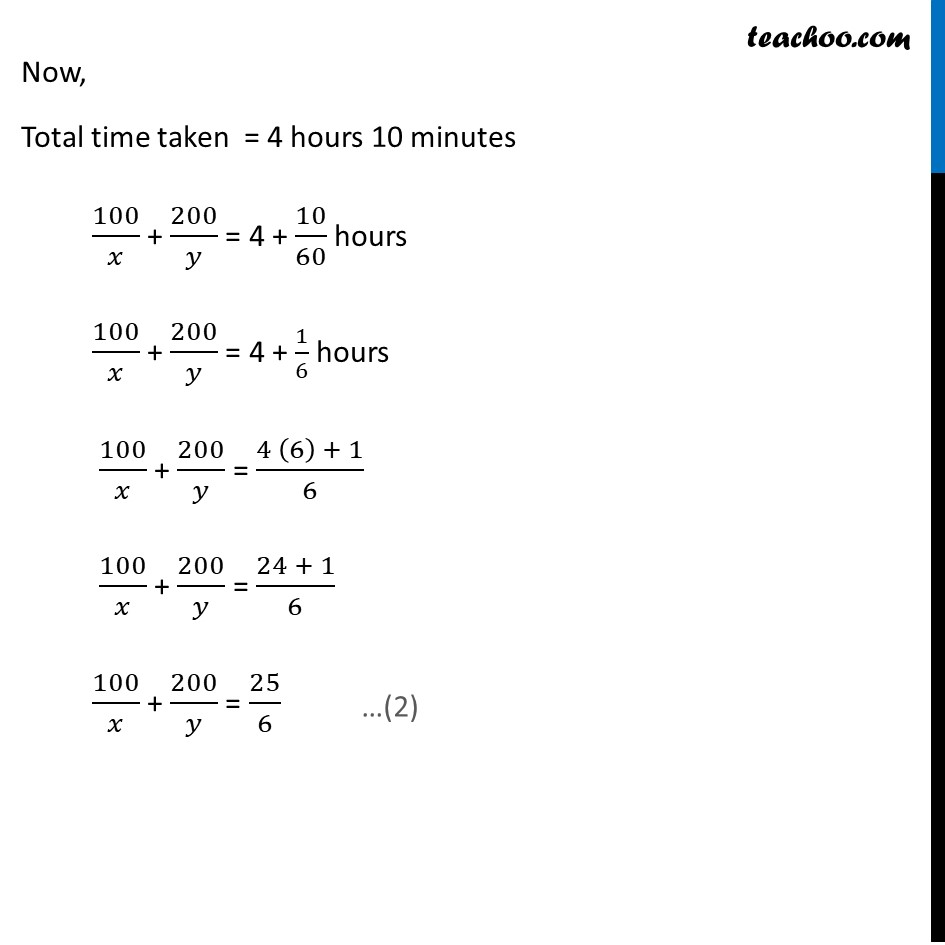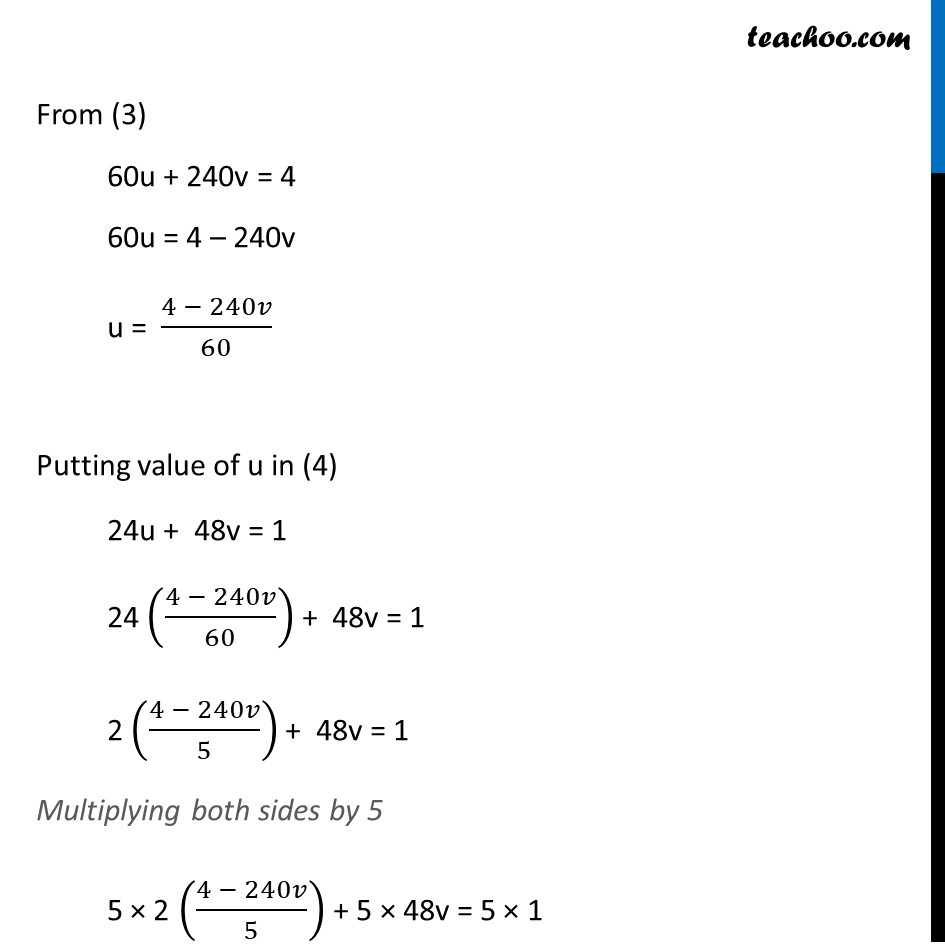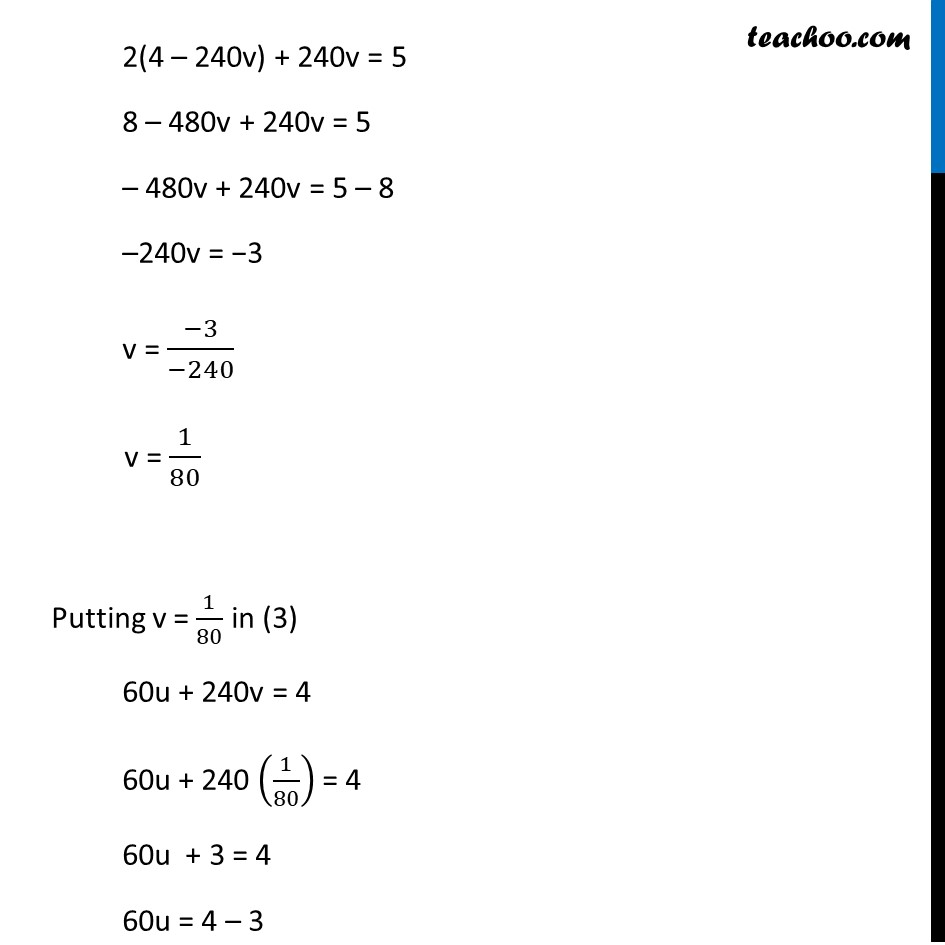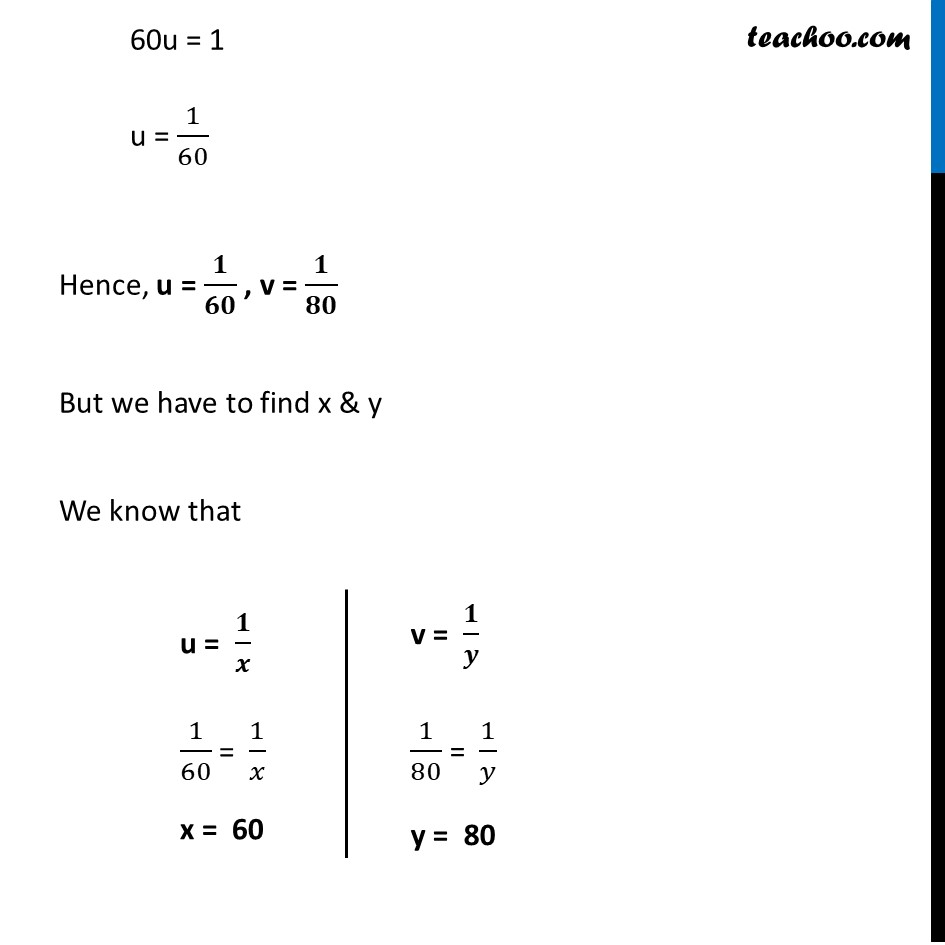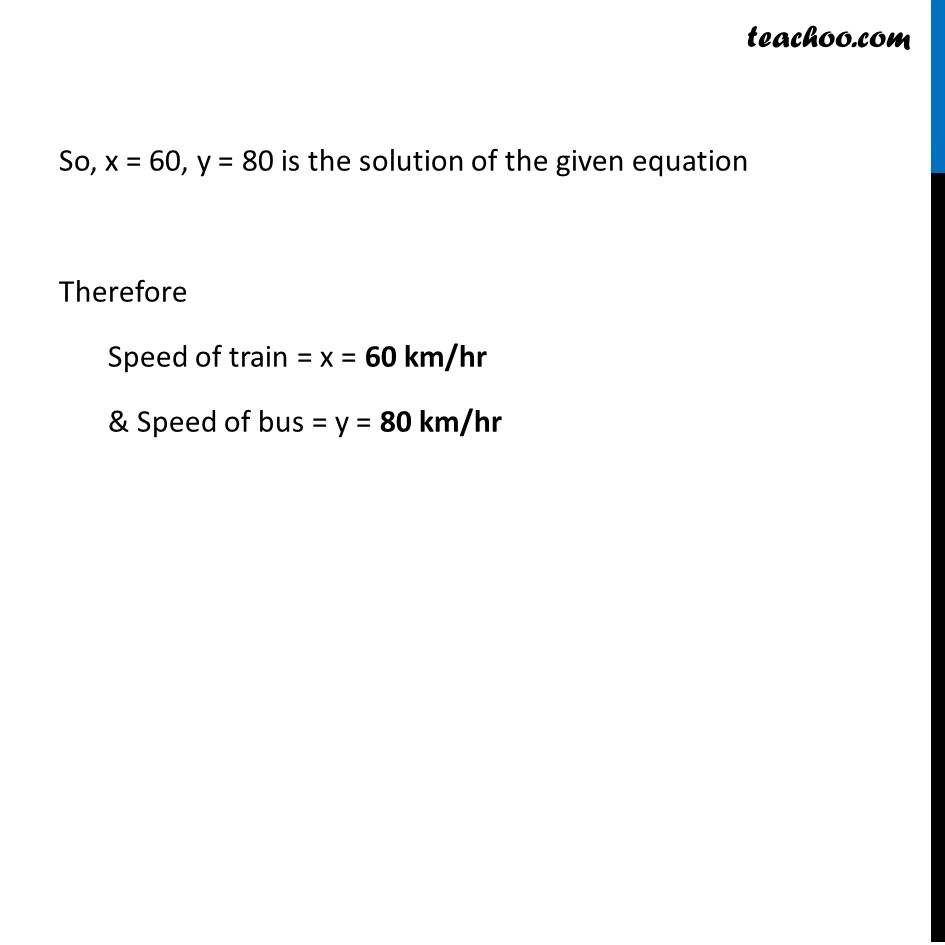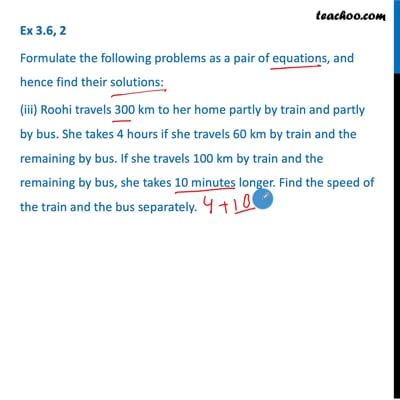This video is only available for Teachoo black users

### Transcript

Ex 3.6, 2 Formulate the following problems as a pair of equations, and hence find their solutions: (iii) Roohi travels 300 km to her home partly by train and partly by bus. She takes 4 hours if she travels 60 km by train and the remaining by bus. If she travels 100 km by train and the remaining by bus, she takes 10 minutes longer. Find the speed of the train and the bus separately. Let speed of train be x km/hr & speed of bus be y km/hr Given that , Roohi travels 300 km to her home partly by train and partly by bus. She takes 4 hours if she travels 60 km by train & the remaining by bus. Train Distance = 60 km Speed = x Time = ? We know that Time = (𝐷𝑖𝑠𝑡𝑎𝑛𝑐𝑒 )/𝑆𝑝𝑒𝑒𝑑 Time = 60/𝑥 Bus Distance = 240 km Speed = y Time = ? We know that Time = (𝐷𝑖𝑠𝑡𝑎𝑛𝑐𝑒 )/𝑆𝑝𝑒𝑒𝑑 Time = 240/𝑦 Now, Total time taken = 4 hours 60/𝑥 + 240/𝑦 = 4 Also, She travels 100 km by train & remaining by bus, she takes 10 min longer. So, she now takes 4 hours, 10 minutes Train Distance = 100 km Speed = x Time = ? We know that Time = (𝐷𝑖𝑠𝑡𝑎𝑛𝑐𝑒 )/𝑆𝑝𝑒𝑒𝑑 Time = 100/𝑥 Bus Distance = 200 km Speed = y Time = ? We know that Time = (𝐷𝑖𝑠𝑡𝑎𝑛𝑐𝑒 )/𝑆𝑝𝑒𝑒𝑑 Time = 200/𝑦 Now, Total time taken = 4 hours 10 minutes 100/𝑥 + 200/𝑦 = 4 + 10/60 hours 100/𝑥 + 200/𝑦 = 4 + 1/6 hours 100/𝑥 + 200/𝑦 = (4 (6) + 1)/6 100/𝑥 + 200/𝑦 = (24 + 1)/6 100/𝑥 + 200/𝑦 = 25/6 So, our two equations are 60/𝑥 + 240/𝑦 = 4 …(1) 100/𝑥 + 200/𝑦 = 25/6 …(2) So, our equations become 60u + 240v = 4 100u + 200v = 25/6 6/25 (100u + 200v) = 1 24u + 48v = 1 Now, we have to solve 60u + 240v = 4 …(3) 24u + 48v = 1 …(4) From (3) 60u + 240v = 4 60u = 4 – 240v u = (4 − 240𝑣)/60 Putting value of u in (4) 24u + 48v = 1 24 ((4 − 240𝑣)/60) + 48v = 1 2 ((4 − 240𝑣)/5) + 48v = 1 Multiplying both sides by 5 5 × 2 ((4 − 240𝑣)/5) + 5 × 48v = 5 × 1 2(4 – 240v) + 240v = 5 8 – 480v + 240v = 5 – 480v + 240v = 5 – 8 –240v = −3 v = (−3)/(−240) v = 1/80 Putting v = 1/80 in (3) 60u + 240v = 4 60u + 240 (1/80) = 4 60u + 3 = 4 60u = 4 – 3 60u = 1 u = 1/60 Hence, u = 𝟏/𝟔𝟎 , v = 𝟏/𝟖𝟎 But we have to find x & y We know that u = 𝟏/𝒙 1/60 = 1/𝑥 x = 60 v = 𝟏/𝒚 1/80 = 1/𝑦 y = 80 So, x = 60, y = 80 is the solution of the given equation Therefore Speed of train = x = 60 km/hr & Speed of bus = y = 80 km/hr